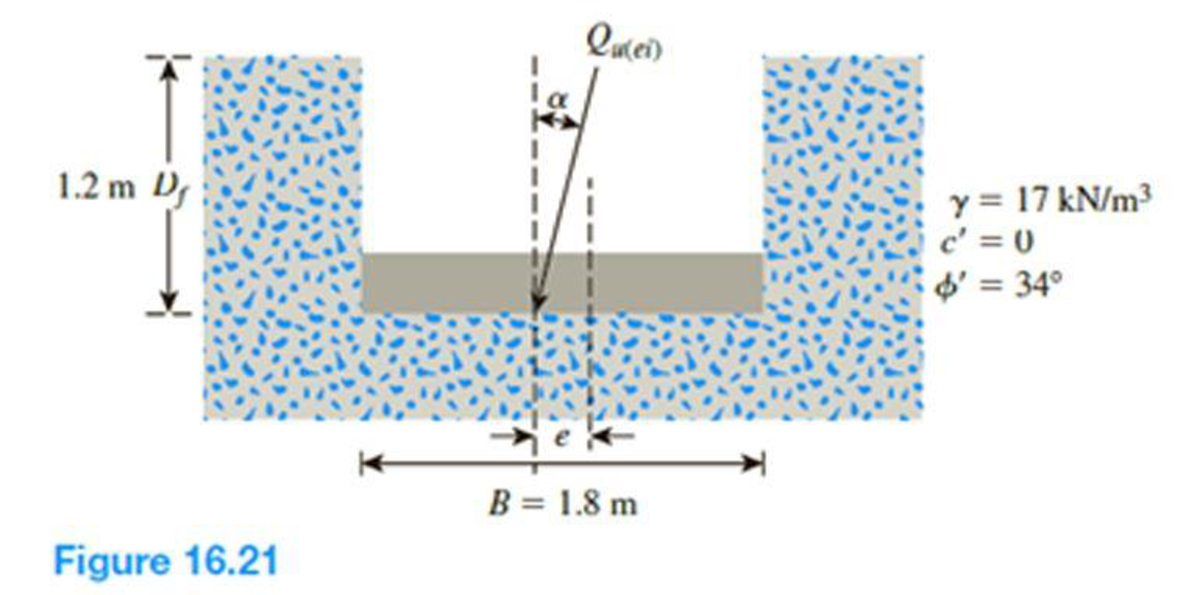Chapter 16, Problem 16.19PPrinciples of Geotechnical Enginee...

9th Edition
Braja M. Das + 1 other
ISBN: 9781305970939

Solutions

Chapter
SectionPrinciples of Geotechnical Enginee...

9th Edition
Braja M. Das + 1 other
ISBN: 9781305970939
Textbook Problem

Figure 16.21 shows a continuous foundation with a width of 1.8 m constructed at a depth of 1.2 m in a granular soil. The footing is subjected to an eccentrically inclined loading with e = 0.3 m, and α = 10°. Determine the gross ultimate load, Qu(ei), that the footing can support using: a. Meyerhof (1963) method [Eq. (16.52)] b. Saran and Agarwal (1991) method [Eq. (16.53)] c. Patra et al. (2012) reduction factor method [Eq. (16.54)](a)

To determine

The gross ultimate load Qu(ei) that the footing can support by using Meyerhof method.

Explanation

Given information:

The unit weight of the soil γ is 17kN/m3.

The value of cohesion c is 0.

The soil friction angle ϕ is 34°.

The location of depth of footing base Df is 1.2 m.

The width of the footing B is 1.8 m.

The value of eccentricity e is 0.3 m.

The inclined angle α is 10°.

Calculation:

Determine the effective width of the footing using the relation.

B=B2e

Substitute 1.8 m for B and 0.3 for e.

B=1.82×0.3=1.2m

For the continuous foundation, all shape factors are equal to one (λcs=λqs=λγs=1.0).

Determine the depth factor λcd using the relation.

λcd=1+0.4tan1(DfB)

Substitute 1.2 m for Df and 1.2 m for B.

λcd=1+0.4(1.21.2)=1.4

Determine the depth factor λqd using the relation.

λqd=1+2tanϕ(1sinϕ)2(DfB)

Substitute 34° for ϕ, 1.2 m for Df, and 1.2 m for B.

λqd=1+2tan34°(1sin34°)2(1.21.2)=1.262

Determine the inclination factor λqi using the relation.

λqi=(1α90°)2

Substitute 10° for α.

λqi=(110°90°)2=0.79

Determine the inclination factor λγi using the relation.

λγi=(1αϕ)2

Substitute 10° for α and 34° for ϕ.

λγi=(110°34°)2=0.498

Determine the ultimate bearing capacity of the soil (qu) using the relation

(b)

To determine

The gross ultimate load Qu(ei) that the footing can support by using Saran and Agarwal method.

(c)

To determine

The gross ultimate load Qu(ei) that the footing can support by using Patra et al. reduction factor method.

Still sussing out bartleby?

Check out a sample textbook solution.

See a sample solution

The Solution to Your Study Problems

Bartleby provides explanations to thousands of textbook problems written by our experts, many with advanced degrees!

Get Started

Compute the interest earned on the deposits made in Problem 20.1.

Engineering Fundamentals: An Introduction to Engineering (MindTap Course List)

What taper turning methods allow use of the lathes power feed?

Precision Machining Technology (MindTap Course List)

What does milky colored ATF indicate?

Automotive Technology: A Systems Approach (MindTap Course List)

What are cryptography and cryptanalysis?

Principles of Information Security (MindTap Course List)

Suggest three typical business situations where referential integrity avoids data problems.

Systems Analysis and Design (Shelly Cashman Series) (MindTap Course List)

What determines the exact temperature of the shielded metal welding arc?

Welding: Principles and Applications (MindTap Course List)

Which Transport layer protocol does PPTP use?

Network+ Guide to Networks (MindTap Course List)